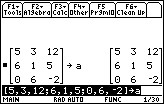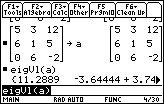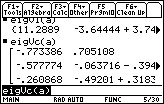# Knowledge Base

## Solution 11714: Calculating the Eigenvalue and Eigenvector of a Matrix on the TI-89 Family, and Voyage™ 200 Graphing Calculators.

### How do I calculate the eigenvalue and eigenvector of a matrix on the TI-89 family, and Voyage 200 graphing calculators?

To calculate the eigenvalue and eigenvector of a matrix on the TI-89 family, and Voyage 200 refer to the instructions below:

Matrix for this example is:
[  5  3  12]
[  6  1  5  ]
[  0  6  -2 ]

To create the matrix, press [HOME] to return to the main screen of the calculator and follow the steps listed below.

1) Press [2nd] [ [ ].
2) Press  [,]  [,]  .
3) Press [2nd] [;].
4) Press  [,]  [,] .
5) Press  [,]  [,] [(-)] .
6) Press [2nd] [ ] ].
7) Press [STO?] [ALPHA] [A] [ENTER].To find the eigenvalue:

1) Press [2nd] [MATH].
2) Select [4:MATRIX] [9:eigVl].
3) Press [ALPHA] [A] [ ) ] [ENTER].To find the eigenvector:

1) Press [2nd] [MATH].
2) Select [4:MATRIX].
3) Press [ALPHA] [A].
4) Press [ALPHA] [A] [ ) ] [ENTER].Please see the TI-89 Titanium / Voyage 200 guidebook for additional information.